Molecular Weight Plastics 001 Molecular Weight Plastics 001

• Slides: 35Molecular Weight Plastics 001Molecular Weight Plastics 001 KEY POINTS: After reviewing the Molecular Weight presentation, students should: • Understand what molecular weight means when dealing with polymers • Understand the effect of molecular weight on material properties • Understand entanglement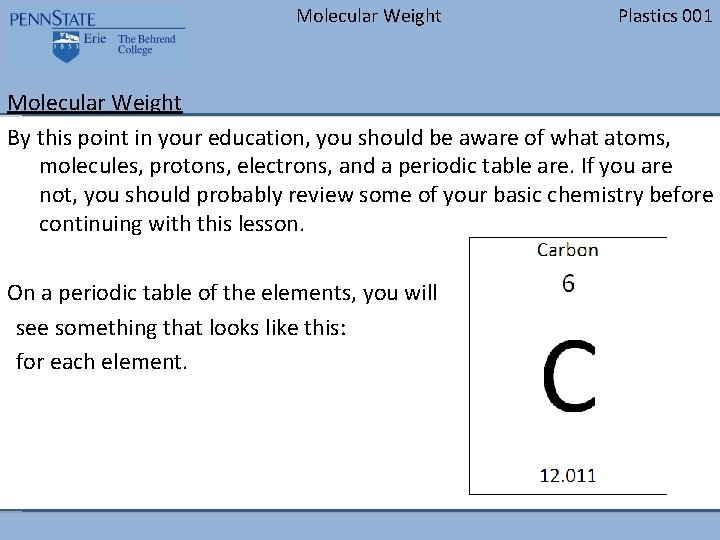Molecular Weight Plastics 001 Molecular Weight By this point in your education, you should be aware of what atoms, molecules, protons, electrons, and a periodic table are. If you are not, you should probably review some of your basic chemistry before continuing with this lesson. On a periodic table of the elements, you will see something that looks like this: for each element.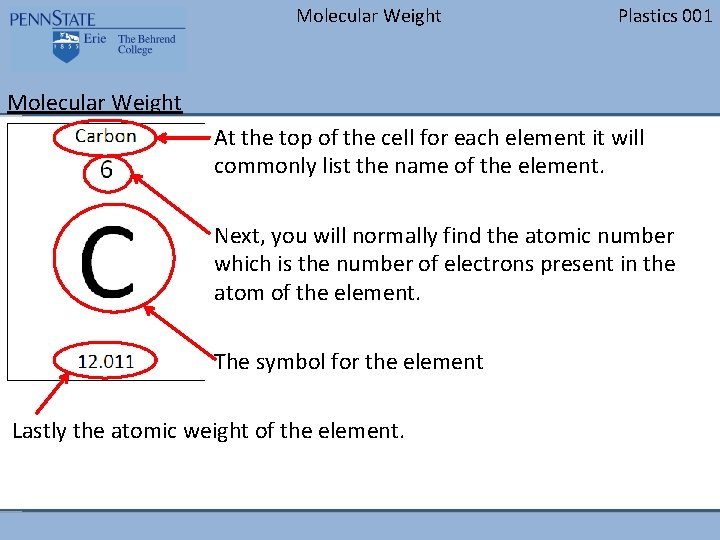Molecular Weight Plastics 001 Molecular Weight At the top of the cell for each element it will commonly list the name of the element. Next, you will normally find the atomic number which is the number of electrons present in the atom of the element. The symbol for the element Lastly the atomic weight of the element.Molecular Weight Plastics 001 Molecular Weight Molecular weight is the sum of the atomic weights of the atoms that make up the molecule. Carbon has an atomic weight of 12. 011 grams/mole. 12 is close enough for what we’re doing A mole is 6. 0221415 × 1023 atoms or molecules This is known as Avogadro's number, it is a count or number of units – like a dozen Because of their extremely long molecules (8, 000 -10, 000 mers long), polymers can have extremely high molecular weights.Molecular Weight Plastics 001 Molecular Weight To put it into perspective: A mole of water (1 oxygen  and 2 hydrogen ) weighs 18 grams – that is barely enough to cover the bottom of a glass. A mole of polypropylene (3 carbons  and 6 hydrogen ) weighs 378, 000 grams (assuming 9, 000 mers have linked up) • That converts to around 830 pounds or almost a full gaylord. Gaylord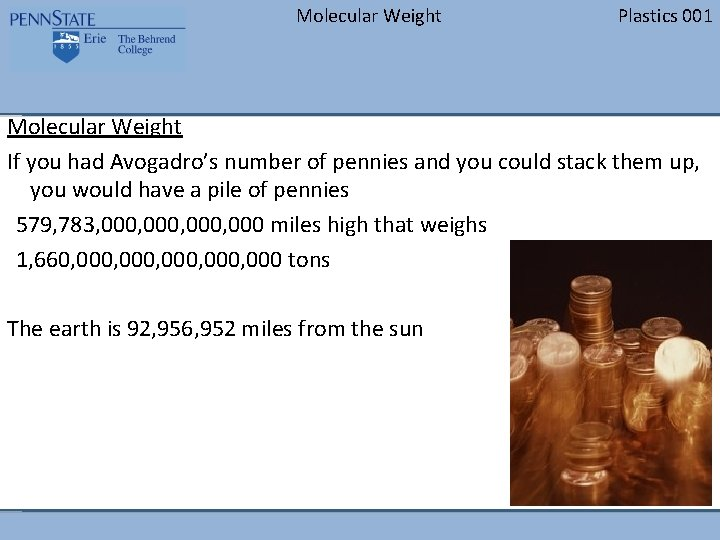Molecular Weight Plastics 001 Molecular Weight If you had Avogadro’s number of pennies and you could stack them up, you would have a pile of pennies 579, 783, 000, 000 miles high that weighs 1, 660, 000, 000 tons The earth is 92, 956, 952 miles from the sun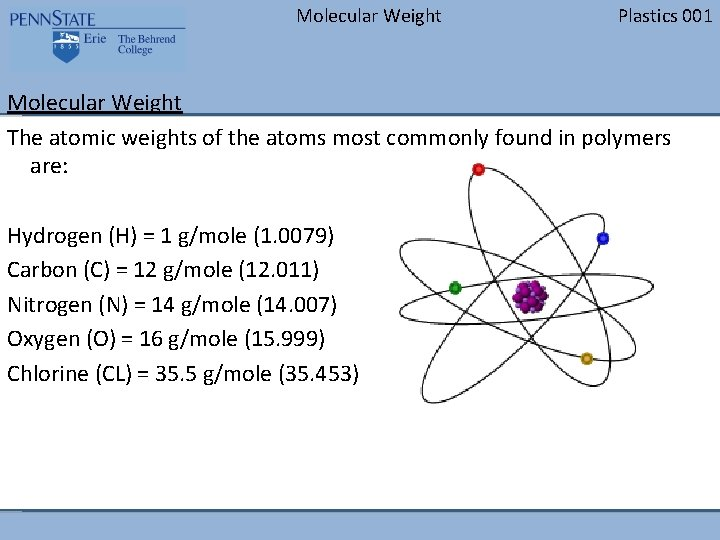Molecular Weight Plastics 001 Molecular Weight The atomic weights of the atoms most commonly found in polymers are: Hydrogen (H) = 1 g/mole (1. 0079) Carbon (C) = 12 g/mole (12. 011) Nitrogen (N) = 14 g/mole (14. 007) Oxygen (O) = 16 g/mole (15. 999) Chlorine (CL) = 35. 5 g/mole (35. 453)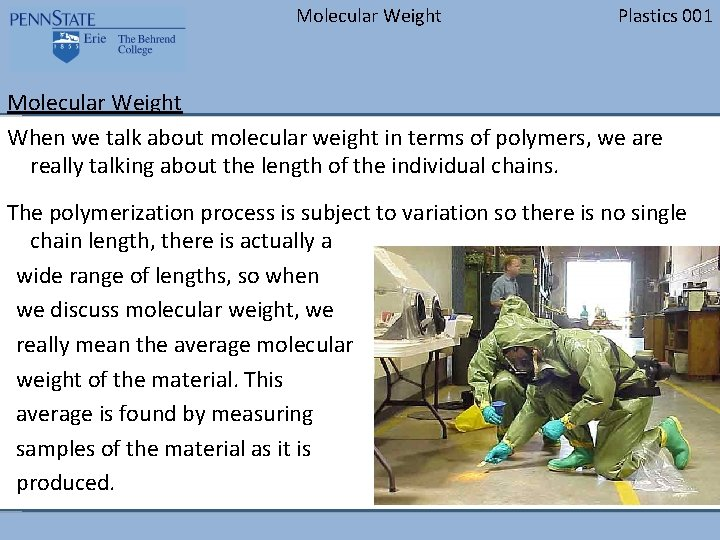Molecular Weight Plastics 001 Molecular Weight When we talk about molecular weight in terms of polymers, we are really talking about the length of the individual chains. The polymerization process is subject to variation so there is no single chain length, there is actually a wide range of lengths, so when we discuss molecular weight, we really mean the average molecular weight of the material. This average is found by measuring samples of the material as it is produced.Molecular Weight Plastics 001 Molecular Weight There are two different categories of molecular weight average that are commonly used: The first is the Number Average Molecular Weight (Mn ) The second is the Weight Average Molecular Weight ( ) Another important aspect of the molecular weight distribution is the shape of the curve.Molecular Weight Plastics 001 Molecular Weight The figure to the right represents a typical molecular weight distribution. The vertical axis represents the number of chains at that length. The horizontal axis represents the different chain lengths. Notice that the longer chains are to the left on the graph and the shorter chains are to the right.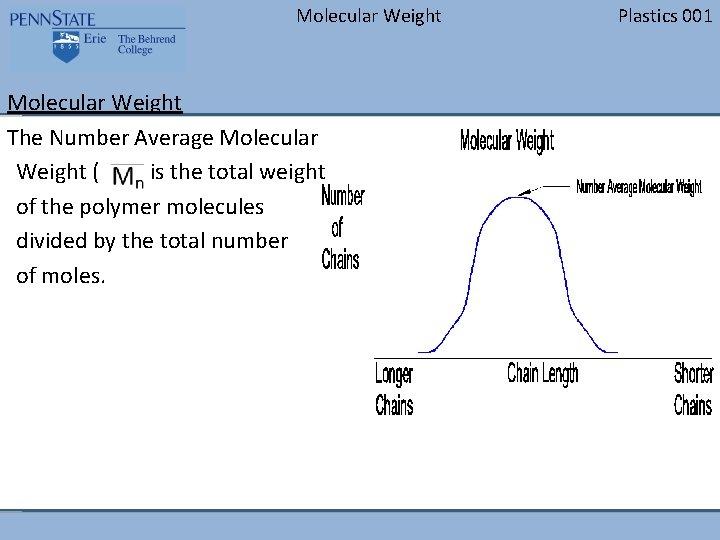Molecular Weight The Number Average Molecular Weight ( ) is the total weight of the polymer molecules divided by the total number of moles. Plastics 001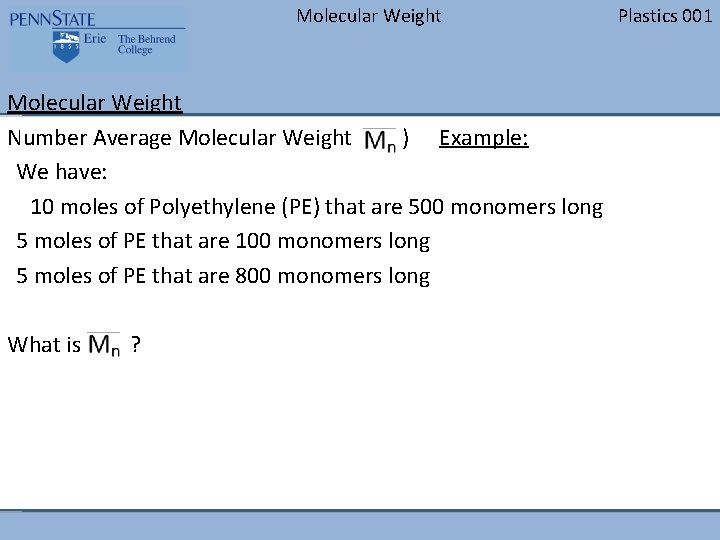Molecular Weight Number Average Molecular Weight ( ) Example: We have: 10 moles of Polyethylene (PE) that are 500 monomers long 5 moles of PE that are 100 monomers long 5 moles of PE that are 800 monomers long What is ? Plastics 001Molecular Weight Number Average Molecular Weight ( ) Example: Each monomer is 2 Carbons and 4 Hydrogens 10 moles X 500 monomers = 5, 000 5 moles X 100 monomers = 500 5 moles X 800 monomers = 4, 000 Total number of moles = 20 (10 + 5) Total number of monomers = 9, 500 What is ? = 475 monomers The average chain is 475 monomers long Plastics 001Molecular Weight The Weight Average Molecular Weight ( ) takes into account that the larger molecules contain a much higher amount of the molecular mass of the polymer. The Weight Average Molecular Weight is almost always higher than the Number Average Molecular Weight ( ). Plastics 001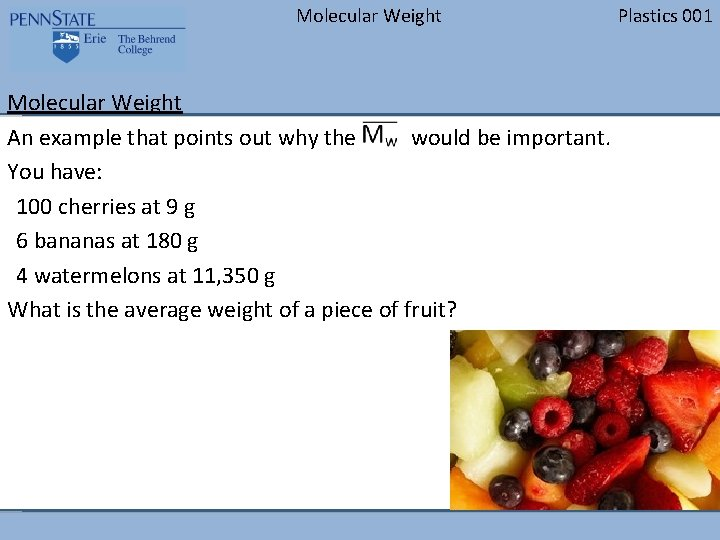Molecular Weight An example that points out why the would be important. You have: 100 cherries at 9 g 6 bananas at 180 g 4 watermelons at 11, 350 g What is the average weight of a piece of fruit? Plastics 001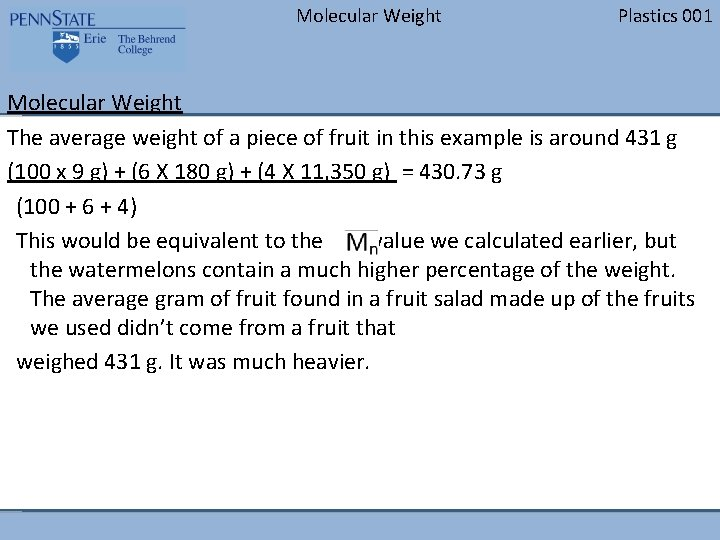Molecular Weight Plastics 001 Molecular Weight The average weight of a piece of fruit in this example is around 431 g (100 x 9 g) + (6 X 180 g) + (4 X 11, 350 g) = 430. 73 g (100 + 6 + 4) This would be equivalent to the value we calculated earlier, but the watermelons contain a much higher percentage of the weight. The average gram of fruit found in a fruit salad made up of the fruits we used didn’t come from a fruit that weighed 431 g. It was much heavier.Molecular Weight Plastics 001 Molecular Weight To calculate the from the example, you need to find the weight fraction of the group. The weight fraction is determined by taking the weight of one of the components and dividing it by the total weight. 100 cherries at 9 g = 900 g 6 bananas at 180 g = 1, 080 g 4 watermelons at 11, 350 g = 45, 400 g The total weight = 47, 380 g The weight fractions are: Cherries: 900/47, 380 = 1. 9 % (The cherries make up 1. 9% of the total weight) Bananas: 1, 080/47, 380 = 2. 3% Watermelons: 45, 400/47, 380 = 95. 8%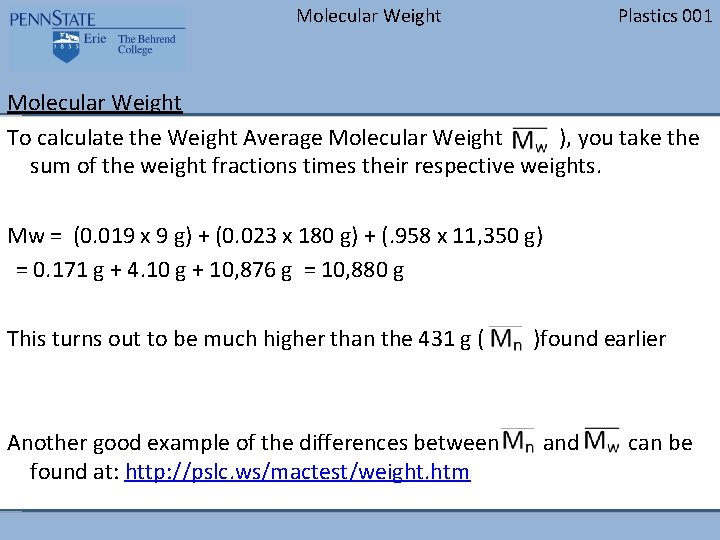Molecular Weight Plastics 001 Molecular Weight To calculate the Weight Average Molecular Weight ( ), you take the sum of the weight fractions times their respective weights. Mw = (0. 019 x 9 g) + (0. 023 x 180 g) + (. 958 x 11, 350 g) = 0. 171 g + 4. 10 g + 10, 876 g = 10, 880 g This turns out to be much higher than the 431 g ( Another good example of the differences between found at: http: //pslc. ws/mactest/weight. htm )found earlier and can be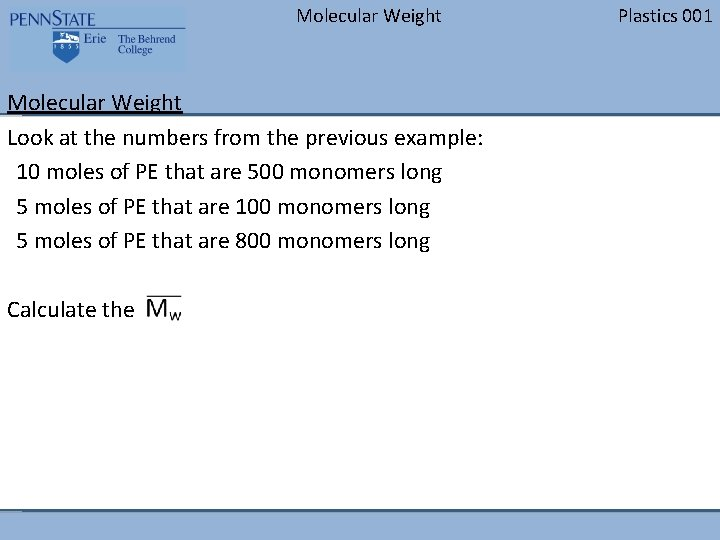Molecular Weight Look at the numbers from the previous example: 10 moles of PE that are 500 monomers long 5 moles of PE that are 100 monomers long 5 moles of PE that are 800 monomers long Calculate the Plastics 001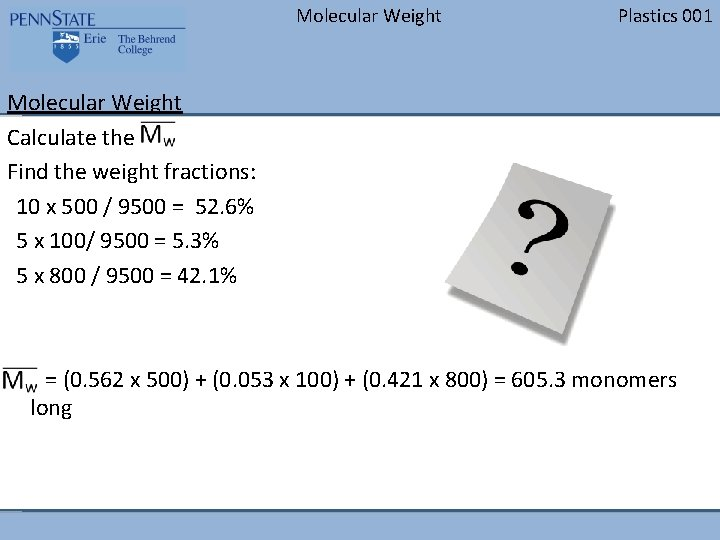Molecular Weight Plastics 001 Molecular Weight Calculate the Find the weight fractions: 10 x 500 / 9500 = 52. 6% 5 x 100/ 9500 = 5. 3% 5 x 800 / 9500 = 42. 1% = (0. 562 x 500) + (0. 053 x 100) + (0. 421 x 800) = 605. 3 monomers long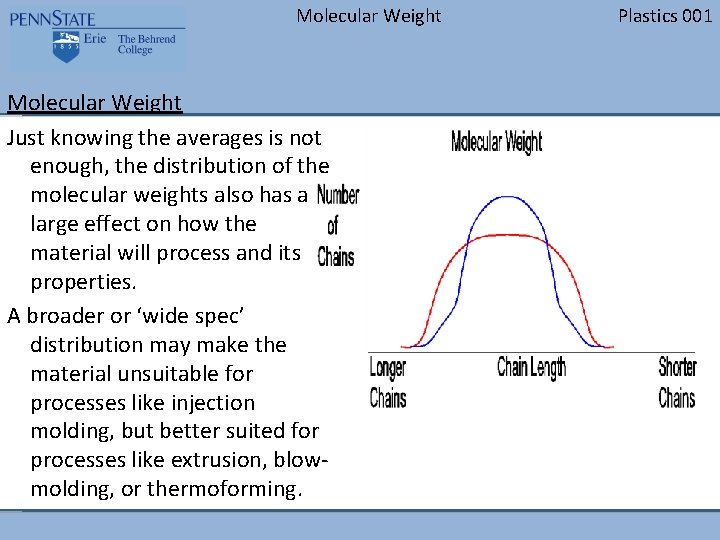Molecular Weight Just knowing the averages is not enough, the distribution of the molecular weights also has a large effect on how the material will process and its properties. A broader or ‘wide spec’ distribution may make the material unsuitable for processes like injection molding, but better suited for processes like extrusion, blowmolding, or thermoforming. Plastics 001Molecular Weight For injection molding grades of material, a narrower distribution is better. When the distribution is narrow, the polymer chains will melt and flow at around the same temperature. The longer the chains, the higher the viscosity or resistance to flow. Plastics 001Molecular Weight When you have a broad or even a bi-modal distribution, the shorter chains melt more quickly and allow some flow, while the longer chains hold the material together. This gives the polymer mixture melt strength which allows it to be used for some of the other processes mentioned other than injection molding. Plastics 001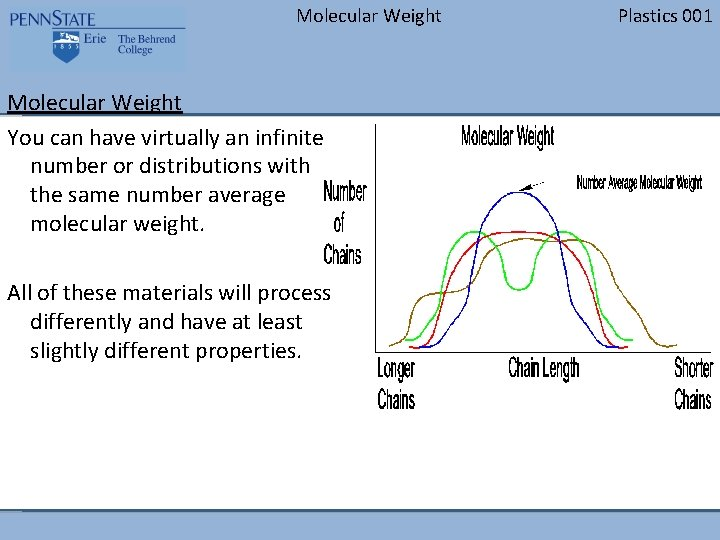Molecular Weight You can have virtually an infinite number or distributions with the same number average molecular weight. All of these materials will process differently and have at least slightly different properties. Plastics 001Molecular Weight Plastics 001 Properties When making polymers, the goal is to make a material with the ideal properties. The longer the molecules (or the higher the molecular weight) the higher the entanglement forces: • Longer hair is harder to get untangled than shorter hairMolecular Weight Plastics 001 Properties Increasing the molecular weight of the material increases many of the properties of the material by increasing the entanglement of the molecules. A higher molecular weight: • Increases the chemical resistance - to a point – It takes more damage to the main chains of the molecules before it will affect the strength of the material – The big loophole to this is if you have a chemical that is very similar to the chemical makeup of the main chain, it will dissolve it much more easily » Like Dissolves Like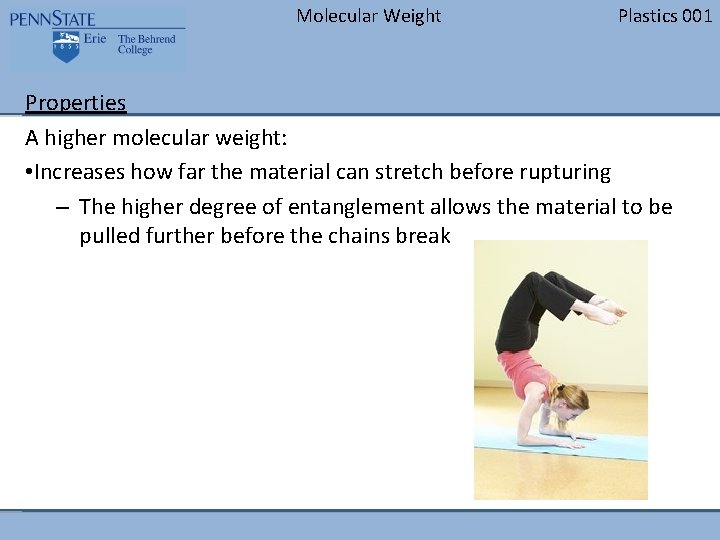Molecular Weight Plastics 001 Properties A higher molecular weight: • Increases how far the material can stretch before rupturing – The higher degree of entanglement allows the material to be pulled further before the chains break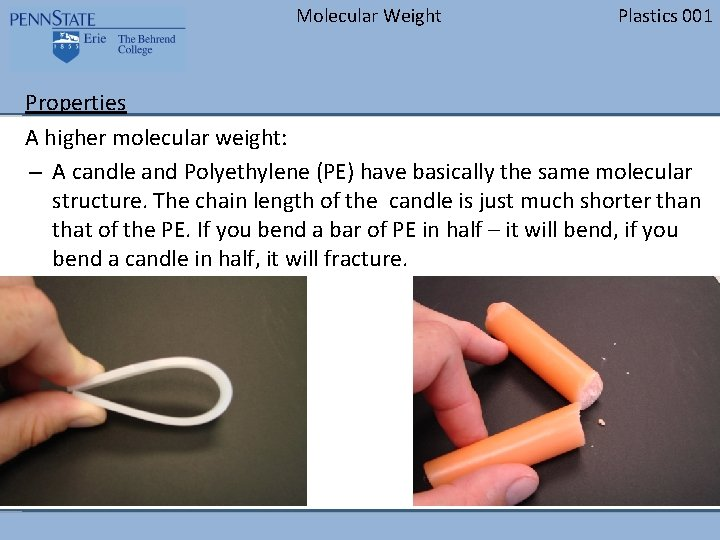Molecular Weight Plastics 001 Properties A higher molecular weight: – A candle and Polyethylene (PE) have basically the same molecular structure. The chain length of the candle is just much shorter than that of the PE. If you bend a bar of PE in half – it will bend, if you bend a candle in half, it will fracture.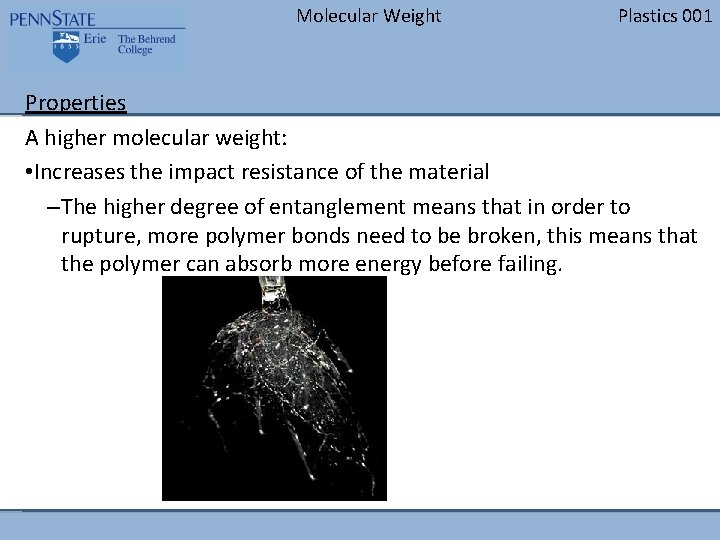Molecular Weight Plastics 001 Properties A higher molecular weight: • Increases the impact resistance of the material –The higher degree of entanglement means that in order to rupture, more polymer bonds need to be broken, this means that the polymer can absorb more energy before failing.Molecular Weight Plastics 001 Properties A higher molecular weight: • Increases the weather resistance of the material – Same type of reasoning behind the increase in chemical resistance, the chains are longer, so they can withstand more damage before the mechanical properties will start to diminish Image by Alex Cheek Creative Commons OAR/ERL/National Severe Storms Laboratory (NSSL)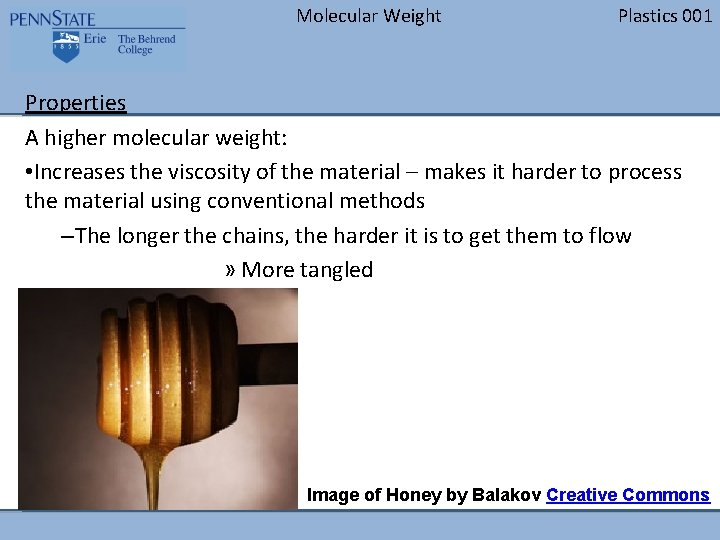Molecular Weight Plastics 001 Properties A higher molecular weight: • Increases the viscosity of the material – makes it harder to process the material using conventional methods –The longer the chains, the harder it is to get them to flow » More tangled Image of Honey by Balakov Creative Commons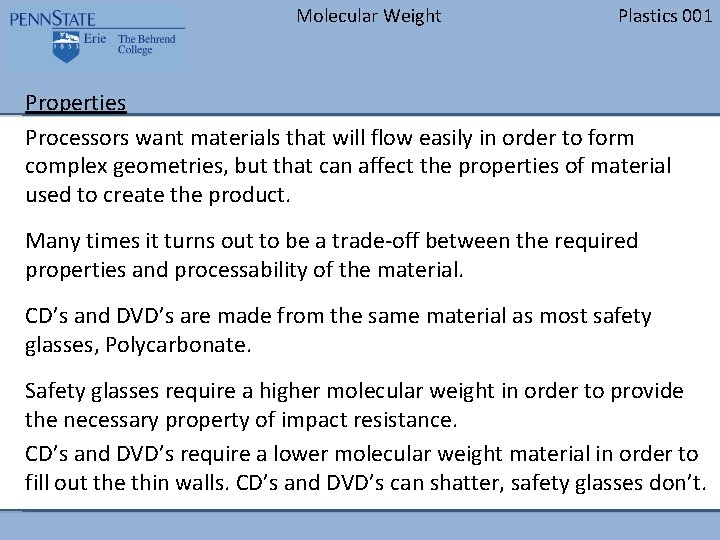Molecular Weight Plastics 001 Properties Processors want materials that will flow easily in order to form complex geometries, but that can affect the properties of material used to create the product. Many times it turns out to be a trade-off between the required properties and processability of the material. CD’s and DVD’s are made from the same material as most safety glasses, Polycarbonate. Safety glasses require a higher molecular weight in order to provide the necessary property of impact resistance. CD’s and DVD’s require a lower molecular weight material in order to fill out the thin walls. CD’s and DVD’s can shatter, safety glasses don’t.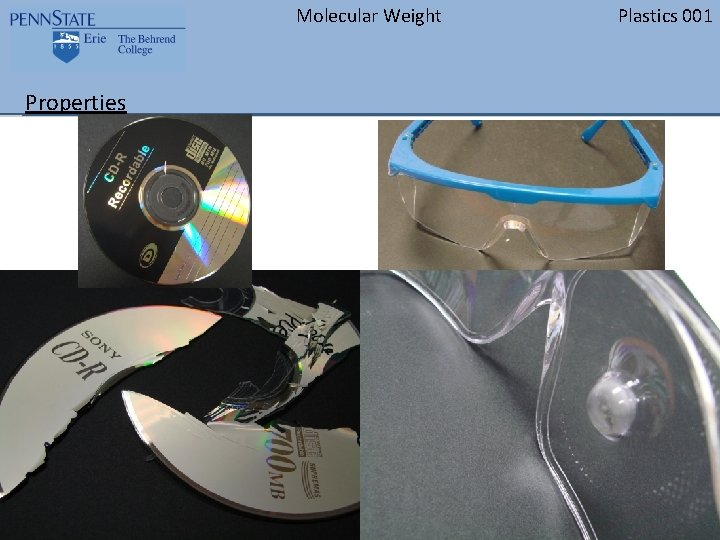Molecular Weight Properties Plastics 001Molecular Weight Questions? Plastics 001